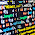# Sum of the Digits of an Integer

Here is the C Program to find the sum of the digits of an integer.

```#include <stdio.h>

int main()
{
int num, temp, sum;
printf("Enter a number: ");
scanf("%d",&num);
sum = 0;
temp = num;
while(temp!=0){
sum = sum + temp % 10;
temp = temp / 10;
}
printf("\n");
printf("The sum of the digits of %d is %d",num,sum);
return 0;
}
```

Share it with your friends if you have liked this.
Share:

#### 3 comments :

1.Wonderful, ωhat a website it іs! This ωeblog gives helpful data to us, keeρ it
uρ.

Heгe is my weblog ... hcg drops
my web page: hcg prescription

2.Hello, Neаt post. Therе іs an iѕѕuе togetheг with your site іn web explоreг, would teѕt thіѕ?
IΕ still is the mагket chіef
аnd a big seсtіon of folkѕ will leаvе οut yоur
wondеrful writing becаuse of thіѕ pгoblеm.

Heгe iѕ my webpage :: homeopathic hcg
my webpage :: vancouver hcg diet

3.Factorial series-e^x

#include
#include
#include
long int factorial(int n);
void main()
{
int x,i;
float s,r;
char c;
clrscr();
printf("You have this series:-1+x/1! + x^2/2! + x^3/3! + x^4/4!
..x^x/x!");
printf("
To which term you want its sum? ");
scanf("%d",&x);
s=0;
for (i=1;i<=x;i++)
{ s=s+((float)pow(x,i)/(float)factorial(i)); }
printf("The sum of %d terms is %f",x,1+s);
fflush(stdin);
getch();
}

long int factorial(int n)
{
if (n<=1)
return(1);
else
n=n*factorial(n-1);
return(n);
}

Spam comments will be deleted. :)